Test: Character Puzzles- 2

# Test: Character Puzzles- 2

Test Description

## 25 Questions MCQ Test CSAT Preparation for UPSC CSE | Test: Character Puzzles- 2

Test: Character Puzzles- 2 for Banking Exams 2023 is part of CSAT Preparation for UPSC CSE preparation. The Test: Character Puzzles- 2 questions and answers have been prepared according to the Banking Exams exam syllabus.The Test: Character Puzzles- 2 MCQs are made for Banking Exams 2023 Exam. Find important definitions, questions, notes, meanings, examples, exercises, MCQs and online tests for Test: Character Puzzles- 2 below.
Solutions of Test: Character Puzzles- 2 questions in English are available as part of our CSAT Preparation for UPSC CSE for Banking Exams & Test: Character Puzzles- 2 solutions in Hindi for CSAT Preparation for UPSC CSE course. Download more important topics, notes, lectures and mock test series for Banking Exams Exam by signing up for free. Attempt Test: Character Puzzles- 2 | 25 questions in 20 minutes | Mock test for Banking Exams preparation | Free important questions MCQ to study CSAT Preparation for UPSC CSE for Banking Exams Exam | Download free PDF with solutions
 1 Crore+ students have signed up on EduRev. Have you?
Test: Character Puzzles- 2 - Question 1

### Which one will replace the question mark ?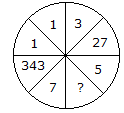Detailed Solution for Test: Character Puzzles- 2 - Question 1

All numbers are cubed,

(7)3 = 343

(1)3 = 1

(3)3 = 27

Similarly, (5)3 = 125.

Test: Character Puzzles- 2 - Question 2

### Which one will replace the question mark ?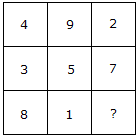Detailed Solution for Test: Character Puzzles- 2 - Question 2

(4 + 9 + 2) = (3 + 5 + 7) = (8 + 1 + 6)

Total in each case = 15.

Test: Character Puzzles- 2 - Question 3

### Which one will replace the question mark ?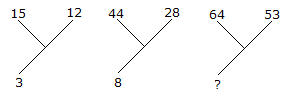Detailed Solution for Test: Character Puzzles- 2 - Question 3

(15 + 12)/9 = 3

and (44 + 28)/9 = 8

Therefore, (64 + 53)/9 = 13.

Test: Character Puzzles- 2 - Question 4

Which one will replace the question mark ?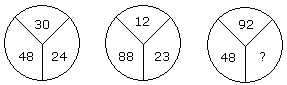Detailed Solution for Test: Character Puzzles- 2 - Question 4

(30 - 24) x 8 = 48

and (23 - 12) x 8 = 88

Therefore, (92 - 86) x 8 = 48.

Test: Character Puzzles- 2 - Question 5

Which one will replace the question mark ?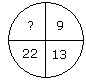Detailed Solution for Test: Character Puzzles- 2 - Question 5

9 + (2)2 = 13

13 + (3)2 = 22

22 + (4)2 = 38.

Test: Character Puzzles- 2 - Question 6

Which one will replace the question mark ?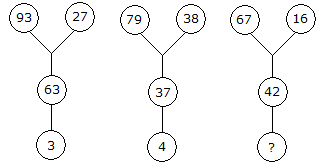Detailed Solution for Test: Character Puzzles- 2 - Question 6

93 - (27 + 3) = 63

79 - (38 + 4) = 37

Therefore, 67 - (16 + X) = 42

X = 9

Test: Character Puzzles- 2 - Question 7

Which one will replace the question mark ?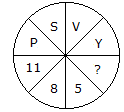Detailed Solution for Test: Character Puzzles- 2 - Question 7

Putting the position of the letters in reverse order

P = 11, S = 8, V = 5 and Y = 2.

Test: Character Puzzles- 2 - Question 8

Which one will replace the question mark ?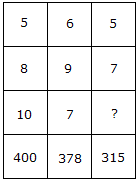Detailed Solution for Test: Character Puzzles- 2 - Question 8

5 x 8 x 10 = 400

and 6 x 9 x 7 = 378

Therefore 5 x 7 x ? = 315

? = 9.

Test: Character Puzzles- 2 - Question 9

Which one will replace the question mark ?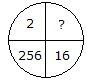Detailed Solution for Test: Character Puzzles- 2 - Question 9

(16)2 = 256

Therefore, (2)2 = 4.

Test: Character Puzzles- 2 - Question 10

Which one will replace the question mark ?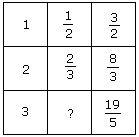Detailed Solution for Test: Character Puzzles- 2 - Question 10

From I row, 1 + (1/2) = 3/2

From II row, 2 + (2/3) = 8/3

From III row, 3 + ? = 19/5

? = (19/5) - 3

? = (4/5).

Test: Character Puzzles- 2 - Question 11

Which one will replace the question mark ?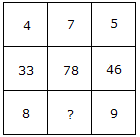Detailed Solution for Test: Character Puzzles- 2 - Question 11

(4 x 8) + 1 = 33

(5 x 9) + 1 = 46

Similarly, (7 x 11) + 1 = 78.

Test: Character Puzzles- 2 - Question 12

Which one will replace the question mark ?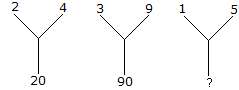Detailed Solution for Test: Character Puzzles- 2 - Question 12

(2)2 + (4)2 = 20

(3)2 + (9)2 = 90

Therefore, (1)2 + (5)2 = 26.

Test: Character Puzzles- 2 - Question 13

Which one will replace the question mark ?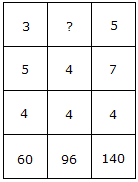Detailed Solution for Test: Character Puzzles- 2 - Question 13

3 x 5 x 4 = 60

and 5 x 7 x 4 = 140

Therefore, 4 x 4 x ? = 96

? = (96/16) = 6.

Test: Character Puzzles- 2 - Question 14

Which one will replace the question mark ?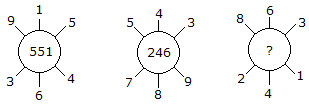Detailed Solution for Test: Character Puzzles- 2 - Question 14

(915 - 364) = 551

(789 - 543) = 246

(863 - 241) = 622.

Test: Character Puzzles- 2 - Question 15

Which one will replace the question mark ?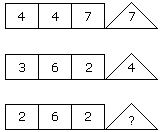Detailed Solution for Test: Character Puzzles- 2 - Question 15

(4 x 7) % 4 = 7

and (6 x 2) % 3 = 4

Therefore, (6 x 2) % 2 = 6.

Test: Character Puzzles- 2 - Question 16

Which one will replace the question mark ?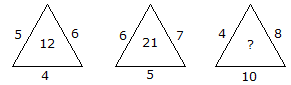Detailed Solution for Test: Character Puzzles- 2 - Question 16

(5 x 6 x 4)/10 = 12

and (6 x 7 x 5)/10 = 21

Therefore (4 x 8 x 10)/10 = 32.

Test: Character Puzzles- 2 - Question 17

Which one will replace the question mark ?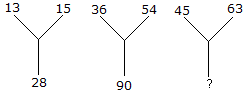Detailed Solution for Test: Character Puzzles- 2 - Question 17

13 + 15 = 28

36 + 54 = 90

Therefore, 45 + 63 = 108.

Test: Character Puzzles- 2 - Question 18

Which one will replace the question mark ?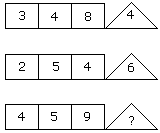Detailed Solution for Test: Character Puzzles- 2 - Question 18

(3 x 4 - 8) = 4

(2 x 5 - 4) = 6

(4 x 5 - 9) = 11.

Test: Character Puzzles- 2 - Question 19

Which one will replace the question mark ?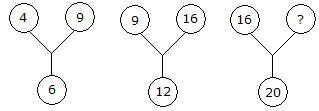Detailed Solution for Test: Character Puzzles- 2 - Question 19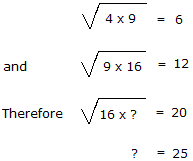Test: Character Puzzles- 2 - Question 20

Which one will replace the question mark ?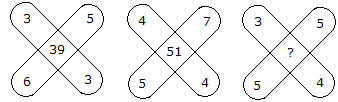Detailed Solution for Test: Character Puzzles- 2 - Question 20

(3 x 3) + (5 x 6) = 39

and (4 x 4) + (5 x 7) = 51

Therefore, (3 x 4) + (5 x 5) = 37.

Test: Character Puzzles- 2 - Question 21

Which one will replace the question mark ?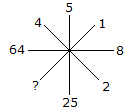Detailed Solution for Test: Character Puzzles- 2 - Question 21

(2)2 = 4

(8)2 = 64

(5)2 = 25

(1)2 = 1.

Test: Character Puzzles- 2 - Question 22

Which one will replace the question mark ?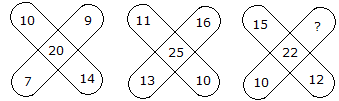Detailed Solution for Test: Character Puzzles- 2 - Question 22

(10 + 9 + 14 + 7)/2 = 20

and (11 + 16 + 10 + 13)/2 = 25

Therefore, (15 + ? + 12 + 10)/2 = 22

Hence 37 + ? = 44

? = 44 - 37

? = 7.

Test: Character Puzzles- 2 - Question 23

Which one will replace the question mark ?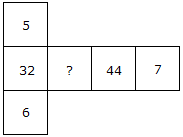Detailed Solution for Test: Character Puzzles- 2 - Question 23

(5 x 6) + 2 = 32

(7 x 6) + 2 = 44

(7 x 5) + 2 = 37.

Test: Character Puzzles- 2 - Question 24

Which one will replace the question mark ?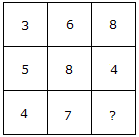Detailed Solution for Test: Character Puzzles- 2 - Question 24

(5 + 3)/2 = 4

and (6 + 8)/2 = 7

Therefore (8 + 4)/2 = 6.

Test: Character Puzzles- 2 - Question 25

Which one will replace the question mark ?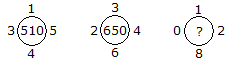Detailed Solution for Test: Character Puzzles- 2 - Question 25

(1)2 + (5)2 + (4)2 + (3)2 = 51 x 10 = 510

and (3)2 + (4)2 + (6)2 + (2)2 = 65 x 10 = 650

Similarly (0)2 + (1)2 + (2)2 + (8)2 = 69 x 10 = 690.

## CSAT Preparation for UPSC CSE

72 videos|64 docs|92 tests
 Use Code STAYHOME200 and get INR 200 additional OFF Use Coupon Code
Information about Test: Character Puzzles- 2 Page
In this test you can find the Exam questions for Test: Character Puzzles- 2 solved & explained in the simplest way possible. Besides giving Questions and answers for Test: Character Puzzles- 2, EduRev gives you an ample number of Online tests for practice

## CSAT Preparation for UPSC CSE

72 videos|64 docs|92 tests